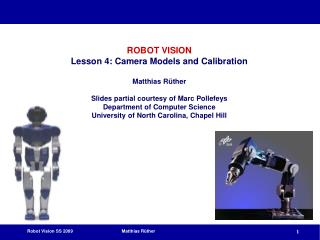DownloadDownload PresentationContent

# Content

Download Presentation## Content

- - - - - - - - - - - - - - - - - - - - - - - - - - - E N D - - - - - - - - - - - - - - - - - - - - - - - - - - -
##### Presentation Transcript

1. ROBOT VISIONLesson 4: Camera Models and CalibrationMatthias RütherSlides partial courtesy of Marc Pollefeys Department of Computer ScienceUniversity of North Carolina, Chapel Hill

2. Content • Camera Models • Pinhole Camera • CCD Camera • Finite Projective Camera • Affine Camera • Pushbroom Camera • Calibration • Inner Orientation • Nonlinear Distortion • Calibration using Planar Targets • Calibration using a 3D Target The Cyclops, 1914 by Odilon Redon

3. Basic Pinhole Camera Model

4. Basic Pinhole Camera Model

5. Basic Pinhole Camera Model

6. Principal Point Offset principal point

7. Principal Point Offset calibration matrix

8. Camera Rotation and Translation

9. CCD Camera

10. non-singular Finite Projective Camera 11 dof (5+3+3) decompose P in K,R,C? {finite cameras}={P3x4 | det M≠0} If rank P=3, but rank M<3, then cam at infinity

11. (pseudo-inverse) Action of Projective Cameras on Points Forward projection (3D -> 2D) D…direction Back-projection (2D -> 3D)

12. arctan(1/s) g 1 When is skew non-zero? for CCD/CMOS, always s=0 Image from image, s≠0 possible (non coinciding principal axis) resulting camera:

13. Moving the Camera Center to Infinity Camera center at infinity Affine and non-affine cameras Definition: affine camera has P3T=(0,0,0,1)

14. Affine Cameras

15. Parallel Projection: Summary canonical representation affine calibration matrix principal point is not defined

16. A Hierarchy of Affine Cameras Orthographic projection (5dof) Scaled orthographic projection (6dof)

17. A Hierarchy of Affine Cameras Weak perspective projection (7dof)

18. A Hierarchy of Affine Cameras Affine camera (8dof) • Affine camera=camera with principal plane coinciding with P∞ • Affine camera maps parallel lines to parallel lines • No center of projection, but direction of projection PAD=0 • (point on P∞)

19. Pushbroom Cameras (11dof) Straight lines are not mapped to straight lines! (otherwise it would be a projective camera)

20. Line Cameras (5dof) Null-space PC=0 yields camera center Also decomposition

21. Camera calibration

22. Problem Statement

23. Basic Equations

24. minimize subject to constraint Basic Equations minimal solution P has 11 dof, 2 independent eq./points • 5½ correspondences needed (say 6) Over-determined solution n  6 points

25. Geometric Error

26. Gold Standard algorithm • Objective • Given n≥6 2D to 2D point correspondences {Xi↔xi’}, determine the Maximum Likelihood Estimation of P • Algorithm • Linear solution: • Normalization: • DLT: • Minimization of geometric error: using the linear estimate as a starting point minimize the geometric error: • Denormalization: ~ ~ ~

27. Exterior Orientation Calibrated camera, position and orientation unkown  Pose estimation 6 dof  3 points minimal (4 solutions in general)

28. Nonlinear Distortion • Radial Component short and long focal length

29. Nonlinear Distortion • Radial Component

30. Correction of radial Distortion Correction of radial distortion • Computing the parameters of the distortion function • Minimize with additional unknowns • Straighten lines • …

31. Nonlinear Distortion • Tangential Component Distortion function:

32. Distortion Workflow (KU wp1) • Project world points: x = P*X • Normalize projected points: xn = K-1 * x • Apply distortion to xn : xd = fdistort(xn) • Denormalize: xp = K * xd